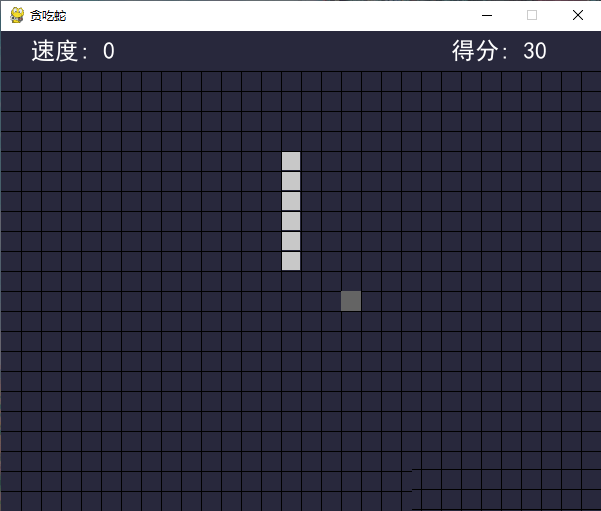# python实现一个简单的贪吃蛇游戏附代码 - Python“贪吃蛇”这个小游戏在编程学习中的常客，因为：

1、蛇怎么表示？

2、蛇怎么移动？

3、如何判定游戏结束？

“蛇”移动超出了游戏区的范围或者碰到了自己就算输了，轴坐标的范围是事先定好的，超出范围很容易判断。那么如何判断碰到自己呢？

`def main():    pygame.init()    screen = pygame.display.set_mode((SCREEN_WIDTH, SCREEN_HEIGHT))    pygame.display.set_caption('贪吃蛇')    light = (100, 100, 100)  # 蛇的颜色    dark = (200, 200, 200)  # 食物颜色    font1 = pygame.font.SysFont('SimHei', 24)  # 得分的字体    font2 = pygame.font.Font(None, 72)  # GAME OVER 的字体    red = (200, 30, 30)  # GAME OVER 的字体颜色    fwidth, fheight = font2.size('GAME OVER')    line_width = 1  # 网格线宽度    black = (0, 0, 0)  # 网格线颜色    bgcolor = (40, 40, 60)  # 背景色    # 方向，起始向右    pos_x = 1    pos_y = 0    # 如果蛇正在向右移动，那么快速点击向下向左，由于程序刷新没那么快，向下事件会被向左覆盖掉，导致蛇后退，直接GAME OVER    # b 变量就是用于防止这种情况的发生    b = True    # 范围    scope_x = (0, SCREEN_WIDTH // SIZE - 1)    scope_y = (2, SCREEN_HEIGHT // SIZE - 1)    # 蛇    snake = deque()    # 食物    food_x = 0    food_y = 0`

` # 初始化蛇    def _init_snake():        nonlocal snake        snake.clear()        snake.append((2, scope_y))        snake.append((1, scope_y))        snake.append((0, scope_y))    # 食物    def _create_food():        nonlocal food_x, food_y        food_x = random.randint(scope_x, scope_x)        food_y = random.randint(scope_y, scope_y)        while (food_x, food_y) in snake:            # 为了防止食物出到蛇身上            food_x = random.randint(scope_x, scope_x)            food_y = random.randint(scope_y, scope_y)    _init_snake()    _create_food()`

`"""贪吃蛇小游戏"""import randomimport sysimport timeimport pygamefrom pygame.locals import *from collections import dequeSCREEN_WIDTH = 600SCREEN_HEIGHT = 480SIZE = 20def print_text(screen, font, x, y, text, fcolor=(255, 255, 255)):    imgText = font.render(text, True, fcolor)    screen.blit(imgText, (x, y))def main():    pygame.init()    screen = pygame.display.set_mode((SCREEN_WIDTH, SCREEN_HEIGHT))    pygame.display.set_caption('贪吃蛇')    light = (100, 100, 100)  # 蛇的颜色    dark = (200, 200, 200)  # 食物颜色    font1 = pygame.font.SysFont('SimHei', 24)  # 得分的字体    font2 = pygame.font.Font(None, 72)  # GAME OVER 的字体    red = (200, 30, 30)  # GAME OVER 的字体颜色    fwidth, fheight = font2.size('GAME OVER')    line_width = 1  # 网格线宽度    black = (0, 0, 0)  # 网格线颜色    bgcolor = (40, 40, 60)  # 背景色    # 方向，起始向右    pos_x = 1    pos_y = 0    # 如果蛇正在向右移动，那么快速点击向下向左，由于程序刷新没那么快，向下事件会被向左覆盖掉，导致蛇后退，直接GAME OVER    # b 变量就是用于防止这种情况的发生    b = True    # 范围    scope_x = (0, SCREEN_WIDTH // SIZE - 1)    scope_y = (2, SCREEN_HEIGHT // SIZE - 1)    # 蛇    snake = deque()    # 食物    food_x = 0    food_y = 0    # 初始化蛇    def _init_snake():        nonlocal snake        snake.clear()        snake.append((2, scope_y))        snake.append((1, scope_y))        snake.append((0, scope_y))    # 食物    def _create_food():        nonlocal food_x, food_y        food_x = random.randint(scope_x, scope_x)        food_y = random.randint(scope_y, scope_y)        while (food_x, food_y) in snake:            # 为了防止食物出到蛇身上            food_x = random.randint(scope_x, scope_x)            food_y = random.randint(scope_y, scope_y)    _init_snake()    _create_food()    game_over = True    start = False  # 是否开始，当start = True，game_over = True 时，才显示 GAME OVER    score = 0  # 得分    orispeed = 0.5  # 原始速度    speed = orispeed    last_move_time = None    pause = False  # 暂停    while True:        for event in pygame.event.get():            if event.type == QUIT:                sys.exit()            elif event.type == KEYDOWN:                if event.key == K_RETURN:                    if game_over:                        start = True                        game_over = False                        b = True                        _init_snake()                        _create_food()                        pos_x = 1                        pos_y = 0                        # 得分                        score = 0                        last_move_time = time.time()                elif event.key == K_SPACE:                    if not game_over:                        pause = not pause                elif event.key in (K_w, K_UP):                    # 这个判断是为了防止蛇向上移时按了向下键，导致直接 GAME OVER                    if b and not pos_y:                        pos_x = 0                        pos_y = -1                        b = False                elif event.key in (K_s, K_DOWN):                    if b and not pos_y:                        pos_x = 0                        pos_y = 1                        b = False                elif event.key in (K_a, K_LEFT):                    if b and not pos_x:                        pos_x = -1                        pos_y = 0                        b = False                elif event.key in (K_d, K_RIGHT):                    if b and not pos_x:                        pos_x = 1                        pos_y = 0                        b = False        # 填充背景色        screen.fill(bgcolor)        # 画网格线 竖线        for x in range(SIZE, SCREEN_WIDTH, SIZE):            pygame.draw.line(screen, black, (x, scope_y * SIZE), (x, SCREEN_HEIGHT), line_width)        # 画网格线 横线        for y in range(scope_y * SIZE, SCREEN_HEIGHT, SIZE):            pygame.draw.line(screen, black, (0, y), (SCREEN_WIDTH, y), line_width)        if game_over:            if start:                print_text(screen, font2, (SCREEN_WIDTH - fwidth) // 2, (SCREEN_HEIGHT - fheight) // 2, 'GAME OVER',                           red)        else:            curTime = time.time()            if curTime - last_move_time > speed:                if not pause:                    b = True                    last_move_time = curTime                    next_s = (snake + pos_x, snake + pos_y)                    if next_s == food_x and next_s == food_y:                        # 吃到了食物                        _create_food()                        snake.appendleft(next_s)                        score += 10                        speed = orispeed - 0.03 * (score // 100)                    else:                        if scope_x <= next_s <= scope_x and scope_y <= next_s <= scope_y                                 and next_s not in snake:                            snake.appendleft(next_s)                            snake.pop()                        else:                            game_over = True        # 画食物        if not game_over:            # 避免 GAME OVER 的时候把 GAME OVER 的字给遮住了            pygame.draw.rect(screen, light, (food_x * SIZE, food_y * SIZE, SIZE, SIZE), 0)        # 画蛇        for s in snake:            pygame.draw.rect(screen, dark, (s * SIZE + line_width, s * SIZE + line_width,                                            SIZE - line_width * 2, SIZE - line_width * 2), 0)        print_text(screen, font1, 30, 7, f'速度: {score // 100}')        print_text(screen, font1, 450, 7, f'得分: {score}')        pygame.display.update()if __name__ == '__main__':    main()`

tips：这个贪吃蛇的游戏还是很简单的，大家可以尝试运行哦。

## 文章评论

• 这篇文章还没有收到评论，赶紧来抢沙发吧~